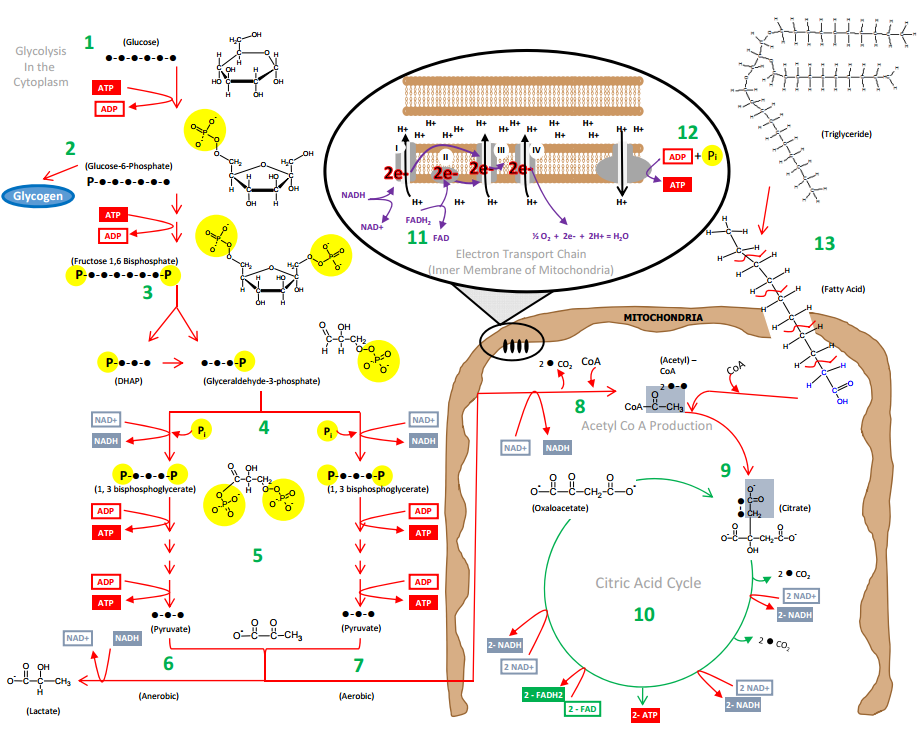7.4

# Reduction-Oxidation Reactions (Redox)

Reduction-oxidation or “redox” reactions are a way to describe the transfer of electrons from one molecular intermediate to another. They are often discussed as half reactions like the oxidation half reaction or the reduction half reaction. Reduction occurs when atoms gain one or more electrons during the reaction which adds a negative charge to the atom. Adding a negative charge (electron) decreases the oxidation number and removing a negative charge (oxidation) increase the oxidation number. The oxidation number of an atom is the charge that the atom would have if the compound was composed of ions. Here are a few rules, the oxidation number of an atom is zero if the atoms all come from the same element. Thus, the atoms in O2 all have an oxidation number of 0. The oxidation number of an ion is equal to the charge of the ion. For the Na+ ion the oxidation number would be +1 and for Cl- it would be -1. Hydrogen is a bit tricky because the oxidation number is +1 if hydrogen in combined with nonmetals (H2O) and -1 if hydrogen is combined with a metal (CaH2).

Thus, a half reaction for a reduction reaction may look something like this:

Cu2+ → Cu

In this example, the initial oxidation number of 2+ for copper is reduced to zero. Cu2+ will be called the oxidizing agent because it is the one getting reduced.

A half reaction for oxidation happens when an atom loses one or more electrons during the chemical reaction so that its oxidation number increases.

Zn → Zn2+

In this example, the initial oxidation number of 0 for zinc is oxidized to 2+. Zn will be called the reducing agent because it is the one getting oxidized.* You can find a detailed description of each metabolic process shown in this image at the end of each section below.  You can also download this image and the complete summary at this link: Metabolism Summary.